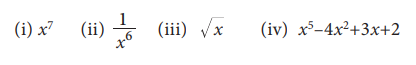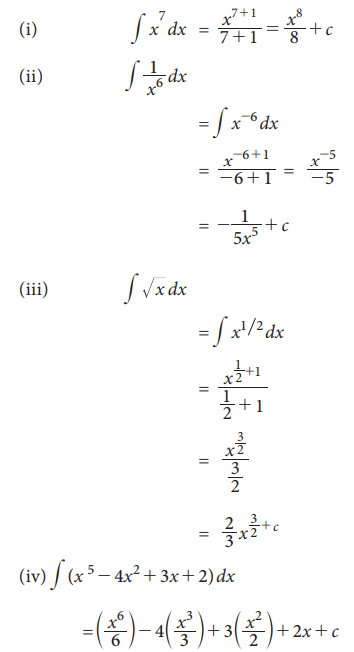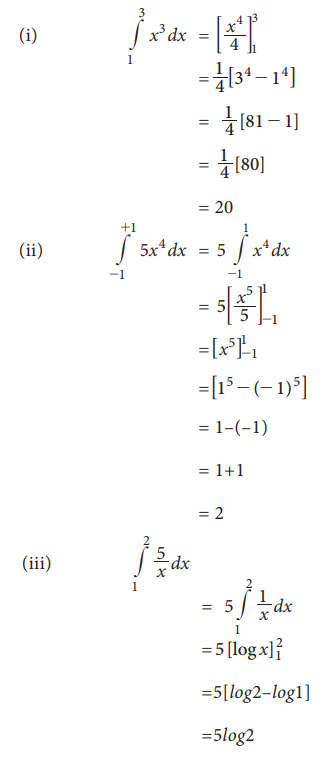Home | | Statistics 11th std | Integration

# Integration

Integration is the reverse process of differentiation. It is also called anti-derivative.

Integration

Integration is the reverse process of differentiation.  It is also called anti-derivative.

Suppose the derivative of x5 is 5x4. Then the integration of 5x4 with respect to x is x5. we use this in symbol as follows:Therefore for the above examples, we usually writeThese integrals are also called improper integrals or indefinite integrals

## Rules and some formulae on integration:### Example 7.21

Integrate the following with respect to x.### Solutions:The above discussed integrals are known as improper integrals or indefinite integrals . For the proper or definite integrals we have the limiting points at both sides. These are called the lower limit and the upper limit of the integral.

This integral ∫(f)dx  is an indefinite integral. Integrating the same function with in the given limits a and b is known as the definite integral. We write this in symbol asIn a definite integral where a is known as the lower limit and b is known as the upper limit of the definite integral. To find the value of definite integral, we do as follows:### Example 7.22

Evaluate the following definite integrals:### Solutions:Tags : Rules, formulae, Solved Example Problems , 11th Statistics : Chapter 7 : Mathematical Methods
Study Material, Lecturing Notes, Assignment, Reference, Wiki description explanation, brief detail
11th Statistics : Chapter 7 : Mathematical Methods : Integration | Rules, formulae, Solved Example Problems